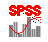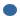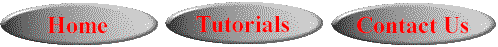# SPSS On-Line Training WorkshopHOME Table of Contents Data Editor Window Syntax  Editor WindowCarl Lee Felix Famoye About Us Chart Editor Window Output Window Overview of Data Analysis Manipulation of Data Analysis of Data Projects & Data Sets Integrate R into SPSS

# Integrating R into SPSSIn this Tutorial:  Preparation for  integrating R SPSS version16 provides an approach to integrate R into SPSS. This is called 'Programmability Extension' underneath the Adds-On Menu. This extension allows unlimited programming capabilities with the SPSS software. This is done through the syntax language to include external programming languages, such as Python, and R. This extension is included with SPSS Base. In this workshop, we shall address the extension to R, a free software that is available through the website, http://www.r-project.org/. You need different versions of R for different versions of SPSS. For SPSS 16, you need R version 2.5 For SPSS 17, you need R version 2.7 For PASW Statistics 18, you need R version 2.8 With the SPSS Programmability Extension, computation not included in SPSS can be done in this external programming language and the results can be saved as an SPSS dataset. In the following, we will demonstrate how to use the Programmability Extension with the program R to conduct a log-linear generalized Poisson regression model. The tasks include: Transfer data from SPSS to R,  Use R to do a Poisson regression and a generalized Poisson regression. Transfer the results back into SPSS.

Preparation for Integrating R

To have access to the extension for R, you need to install SPSS-R Integration Plug-In which is a freeware plug-in. For the current version 16.0 of SPSS, it requires R version 2.5. It is very important that you download and install R version 2.5 and not any other version. Once this is installed, you are ready to take advantage of the R programmability extension. In the later version of SPSS, this restriction may be dropped. Users are advised to consult with the SPSS Help menu to find out the most current restriction.

You need different versions of R for different versions of SPSS.

• For SPSS 16, you need R version 2.5

• For SPSS 17, you need R version 2.7

• For PASW Statistics 18, you need R version 2.8

If you are not familiar with R, you need a crash course in R in order to be an effective user of the extension. To get more information about R, go to the website “the R project for statistical computing”: http://www.r-project.org/ It contains useful information on how to download and install R. There are also various textbooks on R language.

You cannot execute SPSS command syntax from within an R program block. You can have multiple R program blocks separated by SPSS command syntax. Values of variables assigned in one R program block are available in subsequent R programs.

Before you can write an R program, make sure you perform the following tasks:Install SPSS-R Integration Plug-InInstall R version 2.5, if you are running SPSS 16. Make sure you consult with the SPSS Help Menu to find out the required version of R for your SPSS version (Must be Version 16 or later).You are familiar with the R language.

The following commands are needed to begin an R program to be integrated with SPSS:

begin program R.
library (SPSS, version = 16.0)
….......
other R codes and/or SPSS codes
…......
print ( ......... )
end program.When working with begin program R and end program blocks, you will need to use the print function in R to display the output in SPSS viewer. For example, in R environment, the function mean(x) will compute the mean of x and display the value. In this integration, you need to use print(mean(x)).Within a begin program R block, the R functions quit( ) and q( ) will terminate the SPSS session.To read data from SPSS, use the command GetDataFromSPSS. This will read data from SPSS and store it to an R data frame. One can retrieve the cases for all variables or a selected subset of the variables. Variables can be specified by name or an index representing position. The value 0 represents the first variable.To retrieve cases for all variables, use the command:

alldata = spssdata.GetDataFromSPSS( )To retrieve cases for selected variables, use the command:

partdata = spssdata.GetDataFromSPSS( variables = c(“V1,”V2”))To write results to a new SPSS Dataset, use the following commands:

CreateSPSSDictionary(Var1, Var2, … , VarN)

Each argument in the above command is a vector consisting of

-VarName (the variable name)

-VarLabel (the variable label)

-VarType (the variable type; 0 for numeric and an integer equal to the defined length for string variables)

-VarFormat (the variable format; Aw for string and Fw.d for numeric variables)

-VarMeasurement Level (measurement level e.g. “ordinal”, “nominal”, “scale”)

resp = c(“response”, “ ”, 8, “A8”, “nominal”)

int = c(“intercept”, “ ”, 0, “F8.2”, “scale”)

pred = c(“predictor”, “ ”, 0, “F8.2”, “scale”)

dict = spssdictionary.CreateSPSSDictionary(resp, int, pred) spssdictionary.SetDictionaryToSPSS(“results”, dict)

new = data.frame(V1, V2, V3)

spssdata.SetDataToSPSS(“results”, new)

spssdictionary.EndDataStep( )

An Example of Writing R program in SPSS V16 Environment

Generalized Poisson Regression Models

In the generalized linear models clip we addressed the Poisson regression model. For a Poisson regression model, Y is a count response variable with a Poisson distribution. For a generalized Poisson regression model, Y is a count response variable with a generalized Poisson distribution. The mean of Y, mu, is a function of x which is a k-1 dimensional vector of predictor variables. The distribution has f, the dispersion parameter. If f is less than zero, the variance will be less than the mean and the distribution can be used to model under-dispersed data. If f is more than zero, the variance will be more than the mean and the distribution can be used to model over-dispersed data.

In this example, we will use the fabric dataset (see the Data Set page for detail description).  In 1982, Hinde considered a data set on number of faults in rolls of fabric. The dependent (or response) variable ‘faults’ is the number of faults in rolls of fabric and the predictor variable ‘log_length’ is the logarithm of the length of the roll. Under the log-linear mean specification, the Poisson regression model was applied to fit the data.

The Poisson regression model and the generalized Poisson regression model will be fitted to the fabric data by using the R Programmability extension. Note that in the Generalized Linear Model clip we used the Generalized Linear Models to fit the Poisson regression model to the fabric data. However, we noticed that the data is over-dispersed which means that the variance is in excess of the mean relative to the Poisson assumption. Hence, a model like the generalized Poisson regression is more appropriate.

Click on the following clip to learn how to integrate an R program into SPSS. This R program is written for building a generalized Poisson regression model (GPR model). The results from the R program are output for further analysis in SPSS. In this clip, you will find the R code as part of the SPSS syntax for building GPR model. This GPR program is copy righted by Felix Famoye. If you plan to use it for academic research purpose, feel free to download the GPR program. Otherwise, please contact Felix Famoye for permission.©This online SPSS Training Workshop is developed by Dr Carl Lee, Dr Felix Famoye and student assistants Barbara Shelden and Albert Brown , Department of Mathematics, Central Michigan  University. All rights reserved.